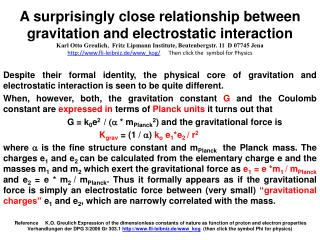DownloadDownload PresentationDespite their formal identity, the physical core of gravitation and electrostatic interaction is seen to be quite differ

# Despite their formal identity, the physical core of gravitation and electrostatic interaction is seen to be quite differ

Download Presentation## Despite their formal identity, the physical core of gravitation and electrostatic interaction is seen to be quite differ

- - - - - - - - - - - - - - - - - - - - - - - - - - - E N D - - - - - - - - - - - - - - - - - - - - - - - - - - -
##### Presentation Transcript

1. A surprisingly close relationship between gravitation and electrostatic interactionKarl Otto Greulich, Fritz Lipmann Institute, Beutenbergstr. 11 D 07745 Jena http://www.fli-leibniz.de/www_kog/ Then click the symbol for Physics Despite their formal identity, the physical core of gravitation and electrostatic interaction is seen to be quite different. When, however, both, the gravitation constant G and the Coulomb constant are expressed in terms of Planck units it turns out that G = k0e2 / (a * mPlanck2) and the gravitational force is Kgrav= (1 / a) ko e1*e2 / r2 where a is the fine structure constant and mPlanckthe Planckmass. The charges e1 and e2 can be calculated from the elementary charge e and the masses m1 and m2 which exert the gravitational force as e1 = e *m1 / mPlanckand e2 = e * m2 / mPlanck. Thus it formally appears as if the gravitational force is simply an electrostatic force between (very small) “gravitational charges” e1 and e2, which are narrowly correlated with the mass.  ReferenceK.O. Greulich Expression of the dimensionless constants of nature as function of proton and electron properties Verhandlungender DPG 3/2006 Gr 303.1http://www.fli-leibniz.de/www_kog (then click the symbol Phi for physics) All fundamental elementary particle masses can be calculated with high accuracy via a simple equation based on the fine structure constant alpha. A simple relationship between particle masses has so far obviously remained unnoticed or at least not become widely accepted. The muon, pion and kaon masses are almost exactly (1.5, 2 and 7 electron masses) / alpha, where alpha is the fine structure constant or coupling constant of quantum electrodynamics.. Based on this the equation m / me = (3M + 1 + d) / alpha has been found to describe the masses of all fundamental elementary particles with an accuracy in the 1% range. Thereby M is a running number from 1 to 12 with an additional island of stability at M = 25 and 26. d is a correction term with the value 0.5 or + / - 1 depending on charm, beauty, strangeness or isospin of the particle. All but 6 particles masses (which have an error between 1 and 2%) , up to the beauty Lambda with 5624 GeV / c2 are calculated with an acuuracy of better than 1 %. This is the most accurate and comprehensive description of particle masses available so far. Reference K.O. GreulichCalculaton of the masses of a l l fundamental elementary particles with an accuracy of approx. 1% 2010 J Mod Phys 1, 300 - 302 http://www.fli-leibniz.de/www_kog (then click the symbol Phi for physics)

2. The Planck particle: the smallest black holeMax Planck had combined G, c and h so that a mass, length, time ….. resultsThe Planck mass is only mass for which ►de Broglie wavelength = Schwarzschild radiusand, as will turn out t h a t mass, for which the „gravitational“ charge = ex axis : Mass in Planck masses √ ħ* c / G= 2.17 * 10 - 8 kg = 4.18 * 1023 electron masses ≈ 1.22×1019 GeV/c2y axis: Diameter in Planck lengths √ħ * G / c3 = 1.61* 10- 35 m

3. The gravitation constant G = ko e2 / (me *mproton * g) can be expressed asG= ko e2 / (a * mPl2) See http://www.fli-leibniz.de/www_kog/ Then click the symbol for Physics K.O. Greulich Expression of the dimensionless constants of nature as function of proton and electron properties Verhandlungen der DPG 3/2006 Gr 303.1 second last slideor verify usingG =6,67 * 10-11 Nm2/kg2 ko=9 *109 Nm2/C2mPl = 2.17645 * 10- 8 kg a =1/137.037 e =1,6 * 10-19 C

4. Gravitation expressed as Coulomb interaction Result of the previous slide G= ko e2 / (a* mPl2)The gravitational force between two masses m1 and m2 then becomesKgrav = G * m1 * m2 / r2Kgrav = ko e2 * m1 * m2 / (a * mPl2* r2)Kgrav = ko*e1* e2 / r2 This is n o t the electrostatic force, it is still gravitationwhere e1 = e *m1 / √ a mPland e2 = e *m2 / √ a mPlor e1 = rPl *m1 / √ a and e2 = rPl *m2 * √ a where rPl is the true specific charge of the Planck particle and rPl/ √ a its specific gravitational charge(8,62 * 10-11 Cb / kg) *

5. Conclusion Karl Otto Greulich, Fritz Lipmann Institute, Beutenbergstr. 11 D 07745 Jena http://www.fli-leibniz.de/www_kog/ Then click the symbol for Physics Gravitation can be completely rewritten as electrostatics when one assigns to matter a very small gravitational charge density ofe/ (mPl√a)= 8,62 * 10-11 Cb / kg = √G / ko where e is the elementary charge, mPl the mass of of the Planck particle, G, ko and a the gravitational, Coulomb and fine structure constantThe actual gravitational charge of a given mass is then m * e / (mPl√ a)So far unexplained is why gravitation is only attractive